Examples

Chapter 13 Class 10 Statistics
Serial order wise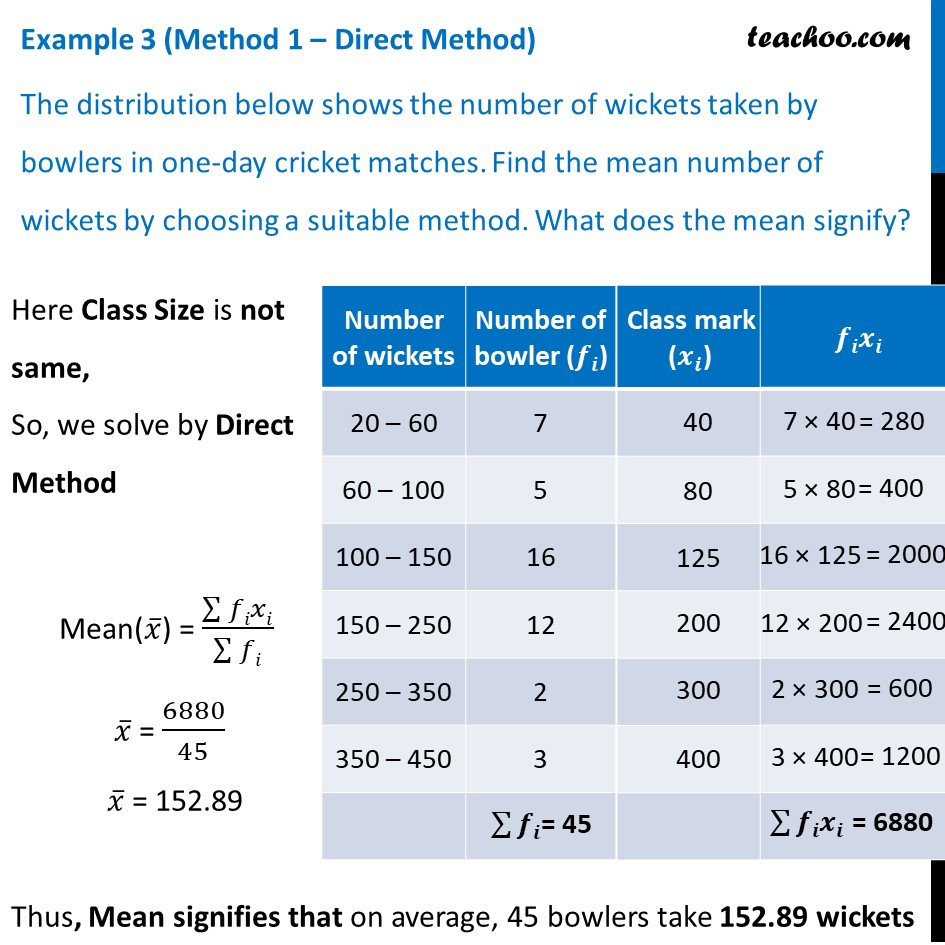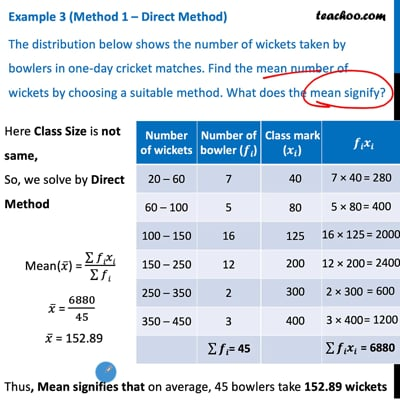This video is only available for Teachoo black users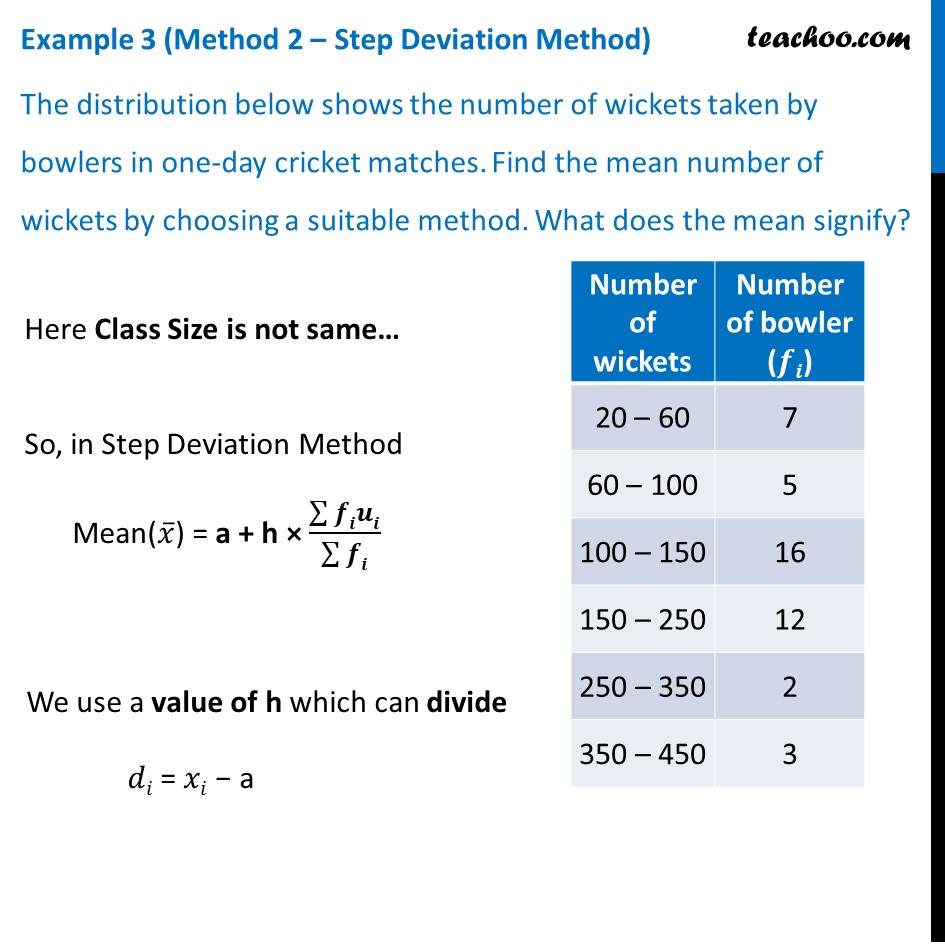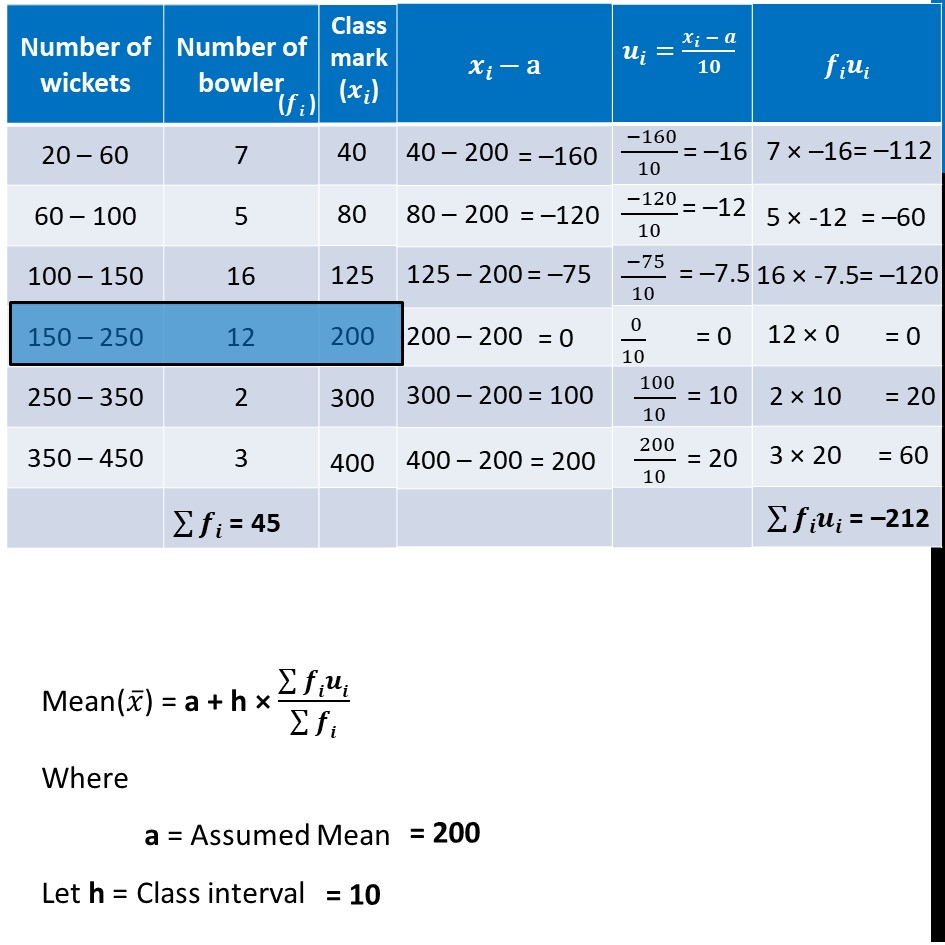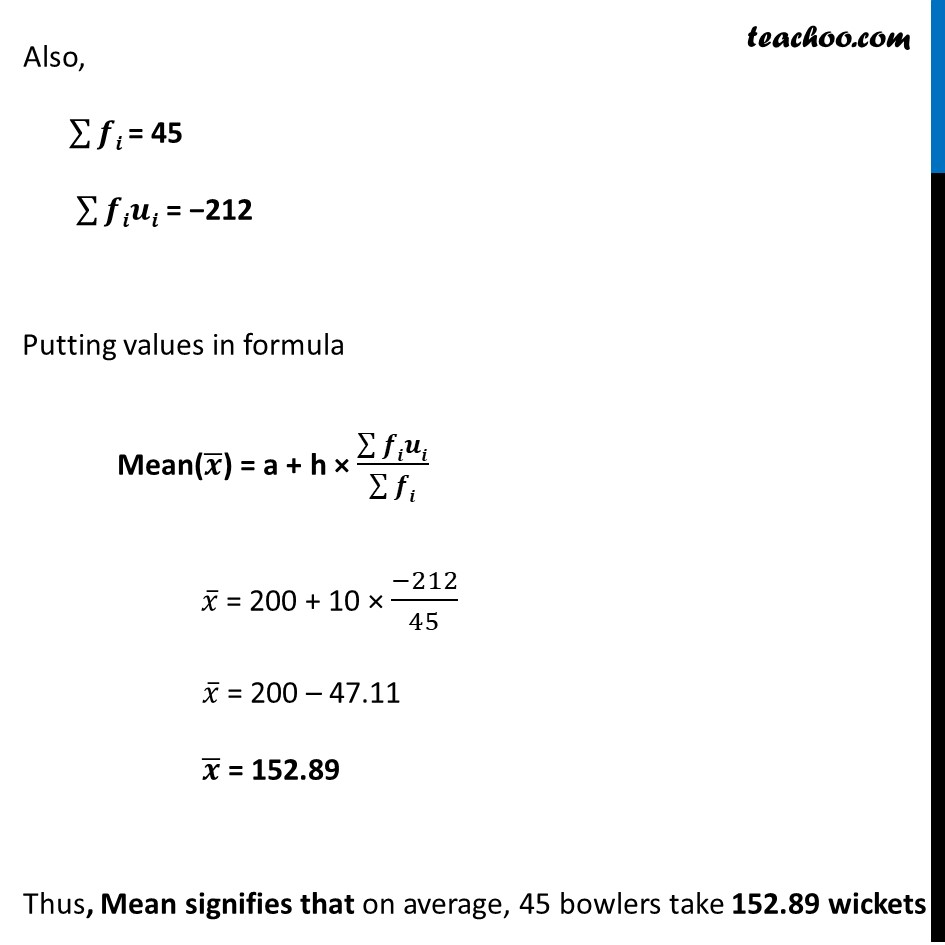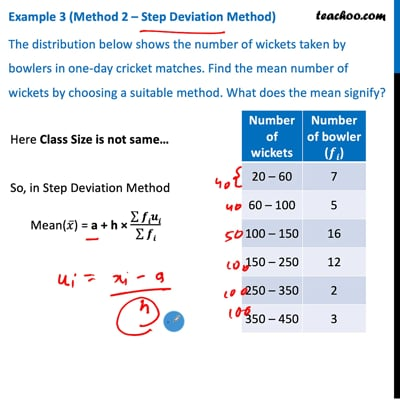This video is only available for Teachoo black users

Learn in your speed, with individual attention - Teachoo Maths 1-on-1 Class

### Transcript

Example 3 (Method 1 – Direct Method) The distribution below shows the number of wickets taken by bowlers in one-day cricket matches. Find the mean number of wickets by choosing a suitable method. What does the mean signify? Here Class Size is not same, So, we solve by Direct Method Mean(𝑥 ̅) = (∑▒𝑓𝑖𝑥𝑖)/(∑▒𝑓𝑖) 𝑥 ̅ = 6880/45 𝑥 ̅ = 152.89 Thus, Mean signifies that on average, 45 bowlers take 152.89 wickets Example 3 (Method 2 – Step Deviation Method) The distribution below shows the number of wickets taken by bowlers in one-day cricket matches. Find the mean number of wickets by choosing a suitable method. What does the mean signify? Here Class Size is not same… So, in Step Deviation Method Mean(𝑥 ̅) = a + h × (∑▒𝒇𝒊𝒖𝒊)/(∑▒𝒇𝒊) We use a value of h which can divide 𝑑𝑖 = 𝑥𝑖 − a Mean(𝑥 ̅) = a + h × (∑▒𝒇𝒊𝒖𝒊)/(∑▒𝒇𝒊) Where a = Assumed Mean Let h = Class interval Also, ∑▒𝒇𝒊 = 45 ∑▒𝒇𝒊𝒖𝒊 = −212 Putting values in formula Mean(𝒙 ̅) = a + h × (∑▒𝒇𝒊𝒖𝒊)/(∑▒𝒇𝒊) 𝑥 ̅ = 200 + 10 × (−212)/45 𝑥 ̅ = 200 – 47.11 𝒙 ̅ = 152.89 Thus, Mean signifies that on average, 45 bowlers take 152.89 wickets